• 使用临近插值法和双线性插值法进行图像处理，将彩色图像缩放之后仍输出彩色图像。
• Write a function Reduce / Expand that has an image as an input and the output should be re-sampled copy of half / twice the width and height of the input image.
• matlab开发-图像处理使用双线性插值缩放动画。本文采用双线性插值法对图像进行正整数因子缩放
• 对BMP图像进行放大及旋转的操作，实现图像成倍数的放大，并在水平面上进行任意角度的旋转。
• 最近邻内插法(nearest)： 原图像中寻找最接近的像素，并把该像素的灰度赋给新像素。双线性内插法(bilinear)：用4个最近邻去估计给定位置的灰度。(最实用)v(x, y) = ax + by + cxy + d;v(x, y)灰度值，a,b,c,d通过四...

内插是在放大、收缩、旋转和几何校正等任务中广泛应用的基本工具。

最近邻内插法(nearest)： 原图像中寻找最接近的像素，并把该像素的灰度赋给新像素。

双线性内插法(bilinear)：用4个最近邻去估计给定位置的灰度。(最实用)

v(x, y) = ax + by + cxy + d;

v(x, y)灰度值，a,b,c,d通过四个最近邻点求得。

双三次内插法(bicubic)：用16个最近邻点赋予给定位置的灰度值.(商业图像编辑程序的标准内插方法).（数字图像处理（P37））

Matlab Keyword: imresize

code：

Image_shrink2_1 = imresize(Image_original,[128 128]);
Image_shrink2_2 = imresize(Image_original,0.5);
Image_enlarge2_nearest = imresize(Image_original,2,'nearest');
Image_enlarge2_bilinear = imresize(Image_original,2,'bilinear');
Image_enlarge2_bicubic = imresize

展开全文matlab 图像放大与缩小 数字图像处理 程序代码
• Matlab——图像缩放（插值法）

万次阅读 多人点赞 2018-07-30 15:49:36
输入原图像以及缩放图像的像素要求（宽度*高度），处理后输出新图像。  我是用matlab来实现scale(input_img,scale_size)函数的，输入图像路径以及要求实现的宽度scale_w和高度值scale_h即可。通过matlab把原...

实验内容：

用双线性内插法实现位深度为8的灰度图像的缩放。

思路：

输入原图像以及缩放后图像的像素要求（宽度*高度），处理后输出新图像。

我是用matlab来实现scale(input_img,scale_size)函数的，输入图像路径以及要求实现的宽度scale_w和高度值scale_h即可。通过matlab把原图像转化为img矩阵，这样矩阵img(a, b)元素的值就是原图像高度为a，宽度为b处的像素值。

设处理前后图像的宽度和高度分别为w、h，scale_w、scale_h。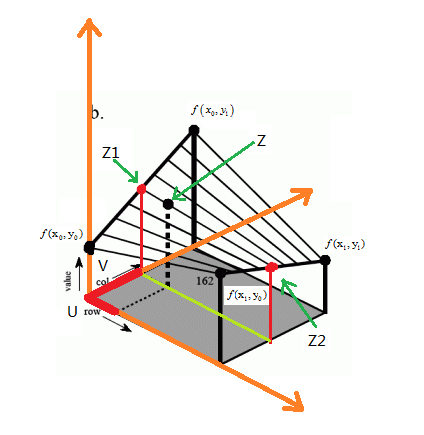如上图所示，row对应的是高度，设为x轴；col对应的是宽度，设为y轴；value对应的是灰度值，设为z轴。

通过缩放比例，可以求得新图像B在(i,j)处对应原图像的点为(x,y)（其中x= i*h/scale_h，y= j*w/scale_w）。

但是这两个值可能为浮点数，而像素中的位置是整数，所以这个点在原图像中对应的可能是“虚”点。所以，我们需

要根据找该“虚”点周围的四个点来进行双线性插值得到新图像的灰度值。我们应该取的是(x,y)邻近的四个像素

(x0,y0) 、(x0,y1) 、(x1,y0) 、(x1,y1)，它们对应的灰度值为f(x0,y0) 、f(x0,y1)、 f(x1,y0)、 f(x1,y1)。

先对x方向进行插值，再对y方向进行插值，计算如下：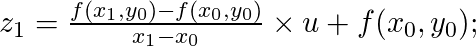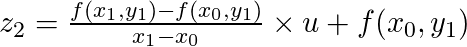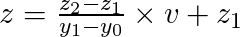反过来，先对y方向：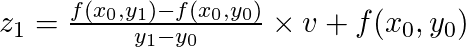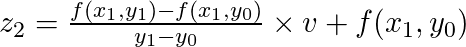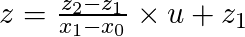显然，邻近像素的x1-x0 = y1-y0 = 1
整理式子得到：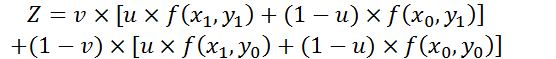这里u、v分别是x、y的小数部分，即u = x - x0; v = y - y0

要注意的是：上述公式img(a,b)中的a和b不能小于1，所以当x和y小于1时需要将其重新赋值为1。

代码：

function output_img = scale(input_img, scale_size)
%Input - input_img is a two-dimensional matrices storing image
%      - scale_size is a tuple of [width, height] defining the spatial resolution of output
%Output - output_img is the same as input_img

[h,w] = size(img); %获取行和列，即原图的高度和宽度

scale_w = scale_size(1); %根据输入获得缩放后的新宽度
scale_h = scale_size(2); %根据输入获得缩放后的新高度
output_img = zeros(scale_h, scale_w); %初始化

for i = 1 : scale_h         %缩放后的图像的（i,j）位置对应原图的（x,y）
for j = 1 : scale_w
x = i * h / scale_h;
y = j * w / scale_w;
u = x - floor(x);
v = y - floor(y); %取小数部分

if x < 1           %边界处理
x = 1;
end

if y < 1
y = 1;
end

%用原图的四个真实像素点来双线性插值获得“虚”像素的像素值
output_img(i, j) = img(floor(x), floor(y)) * (1-u) * (1-v) + ...
img(floor(x), ceil(y)) * (1-u) * v + ...
img(ceil(x), floor(y)) * u * (1-v) + ...
img(ceil(x), ceil(y)) * u * v;
end
end

imwrite(uint8(output_img), '../output_img.png'); %保存处理后的图像
imshow(input_img); %显示原图
figure,imshow(uint8(output_img)) %显示处理后的图像

运行时若图片在代码上一级目录，则输入scale('../a.png', [450,300]);

效果图：

原图为384*256：缩小成192*128：放大成450*300：缩放成500*200：//

展开全文Matlab 数字图像处理 图像缩放 插值法
• 利用matlab进行图像的一些操作处理 缩放和旋转功能的实现
• 图像缩放 A = umesize(I, scale). I:原图像 scale:>1,放大；<1,缩小 A：缩放后的图像 I=imread('C:\Users\14372\Desktop\Music_pictures\图片\63567947_p0.jpg'); A=imresize(I,0.5); figure; imshow(I);...
• 图像的缩放
A = imresize(I, scale).
I:原图像
scale:>1,放大；<1,缩小
A：缩放后的图像
A=imresize(I,0.5);

figure;
imshow(I);title('原图');
figure;
imshow(A);title('缩小0.5倍');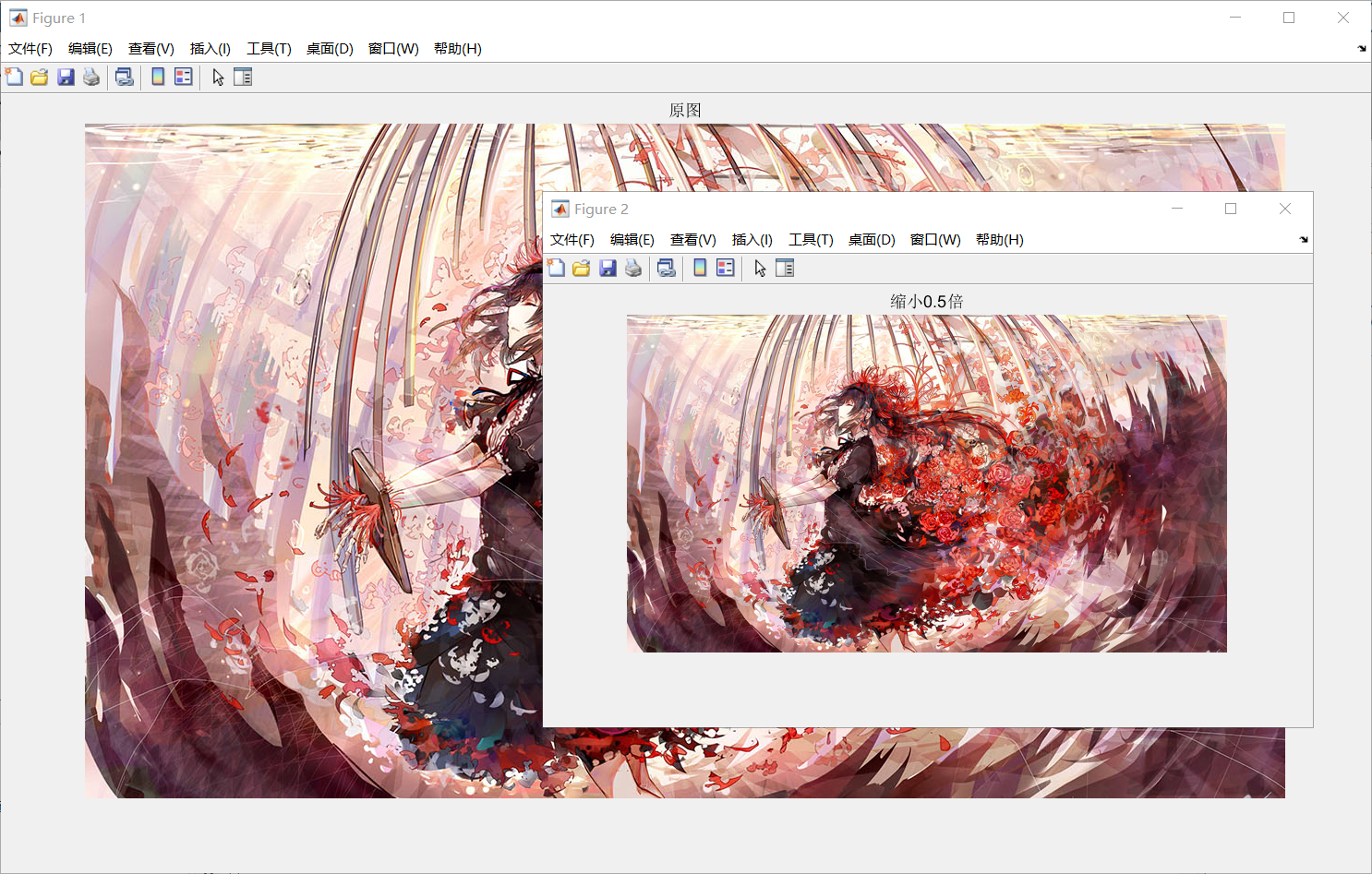• 图像的旋转
A=imrotate(I,angle);
I:原图像
angle:逆时针旋转的角度
A：旋转后的图像
A=imrotate(I,15);

figure;
subplot(1,2,1);imshow(I);title('原图');
subplot(1,2,2);imshow(A);title('逆时针旋转15°');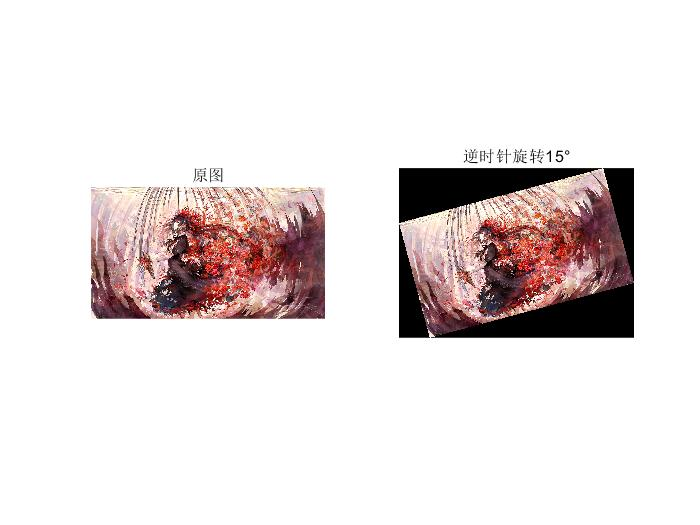• 图像的修剪

https://blog.csdn.net/justforacm/article/details/80664025

A=imcrop(I,[200,200,400,200 ]);

figure;
subplot(1,2,1);imshow(I);title('原图');
subplot(1,2,2);imshow(A);title('裁剪图');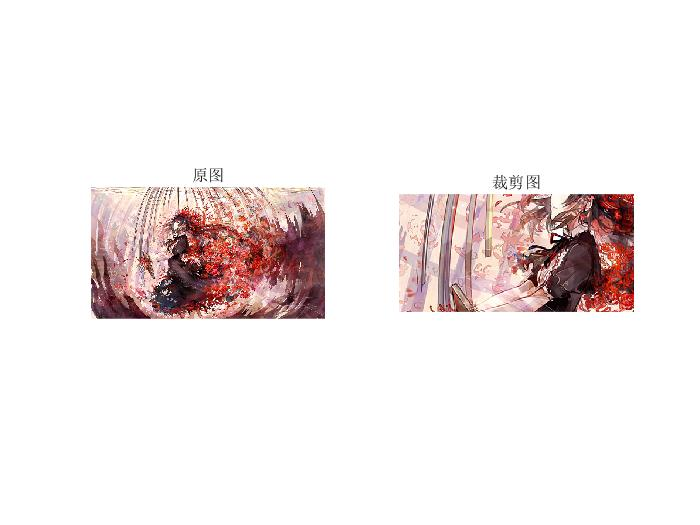展开全文matlab
• matlab实现图像的缩放

万次阅读 2019-07-03 19:07:09
matlab实现图像缩放 缩放同样是仿射变换的一种特例，它接受水平和垂直两个方向的缩放值。 matlab 实现水平，垂直，水平垂直缩放： clear all close all clc img = imread('test.jpg'); %读取输入图片的...

matlab实现图像的缩放

缩放同样是仿射变换的一种特例，它接受水平和垂直两个方向的缩放值。matlab 实现水平，垂直，水平垂直缩放：

clear all
close all
clc
[h,w,d] = size(img); %获取行和列，即原图的高度和宽度
xscale = 3;
yscale = 3;
ht = floor(h./xscale);
wt = floor(w./yscale);
%zoo = zeros(ht,w,d);
for i = 1:ht
for j = 1:w
% for k = 1:d
%zoo(i,j,d) = img(floor(i*xscale),j,d);
% end
zooh(i,j) = img(floor(i*xscale),j);
end
end

for i = 1:h
for j = 1:wt
zoow(i,j) = img(i,floor(j*xscale));
end
end

for i = 1:ht
for j = 1:wt
zoohw(i,j) 
展开全文缩放 matlab实现缩放
• %%%%%——缩放 simg = imresize(sourceImg,1.5); %%%%%%%%%%%%%%%%%%%%%%%%%%%%%%%%%%%%%%%%%%%%%%%%%%%%%%%%%%%%%%%%%%%%%——直方图均衡——%%%%%%%%%%%%%%%%%%%%%%%%%%%%%%%%%%%%%%%%%%%%%%%%%%%%%%%%%% %%%%%...matlab 图像识别
• MATLAB图像处理的实例

千次阅读 2020-11-20 17:02:53
MATLAB实现的图像处理基础的案例，如HSI,直方图统计等。算法 图像处理 matlab 计算机视觉
• 由于在matlab中较多的图像处理函数支持对灰度图像进行处理，故对图像进行灰度转化十分必要。可利用rgb2gray（X）函数对其他图像进行灰度图像的转化。 转化实例如下： 实现程序段如下： % --- Executes on button ...
• Matlab图像缩放 Matlab实现函数代码如下： clc I=rgb2gray(imread('Campus-scenery2sk.JPG')); figure,imshow(I); title('原图'); reduceI=imresize(I,0.5); %原图像I缩小0.5倍 figure,imshow(reduceI); title('...matlab
• （1）图像基本操作：不同格式（大于3种）图像的读入与...（3）图像恢复：几何操作（如旋转、缩放、投影校正等）、模糊恢复（如运动模糊消除等，加分项）；（4）图像合成（加分项）：实现换头、换背景、图像拼接等功能。
• MATLAB图像处理实例详解作者：杨丹，赵海滨，龙哲出版时间：2013年版内容简介《MATLAB图像处理实例详解》全面、系统地介绍了MATLAB在数字图像处理中的各种技术及应用。本书对图像处理的基础概念做了必要交代，重点给...
• 基于matlab GUI灰度+缩放+存储 二、源代码 function varargout = GUI_TEST(varargin) % GUI_TEST MATLAB code for GUI_TEST.fig % GUI_TEST, by itself, creates a new GUI_TEST or raises the existing % singleton...matlab
• 使用matlab对图像进行缩放

千次阅读 2018-03-23 10:46:10
参考：https://www.cnblogs.com/zangyu/p/5395504.html对图像进行固定大小的缩放clear; clc; ％清除以前的数据 folderName = dir('train'); ％显示train文件夹下的文件和文件夹 for i = 1 : length(folderNa...Matlab 图像处理
• 利用matlab对输入的图像（转换为灰度图像）进行图像变换
• MATLAB实现图像的放大和缩放

千次阅读 2021-11-02 22:26:20
t=imread('图像处理.png'); [m,n,dep]=size(t); rm=3630; rn=2355; rt=zeros(rm,rn,dep); for i=1:rm for j=1:rn x=i*m/rm; y=j*n/rn; u=x-floor(x); v=y-floor(y); if x<1 x=1; end if y<1 y=1; end rt(i,j,:...matlab 图像处理 计算机视觉
• MATLAB图像处理工具箱提供了3种插值方法：第一种是最近邻插值（nearest neighbor interpolation），最近邻插值的输出像素值等于输入图像中与其最临近的像素点的值；第二种是双线性插值（bilinear interpolation），...图像处理
• 学习一门技术最好的方式就是阅读官方文档,可以查看MATLAB官方文档图像的读取和展示图像MATLAB中的存储格式MATLAB能够处理的数字图像分为三种:二值图像,灰度图像,彩色图像. 二值图像MATLAB中以一个矩阵存储,矩阵...
• Matlab图像处理函数大全（建议收藏）

千次阅读 多人点赞 2020-12-11 20:32:01
图像去模糊第10章： 线性滤波和变换第11章： 形态学操作第12章： 图像的块和邻域处理第13章： 颜色映射表和色彩空间第14章： 其他常用函数 第1章： 图像显示与图像文件输入输出函数 1.1图像显示函数 1.1.1 immovie...matlab 函数 图像处理
• Matlab实现图像的比例缩放

万次阅读 多人点赞 2018-05-10 22:35:49
以灰度图像circuit.tif为例，利用Matlab图像处理工具箱中的imresize函数对图像进行比例缩放变换。要求：创建4个figure窗口（不可以用subplot，显示不出来放大效果），分别用于显示原始图像、等比例放大1.5倍后的图像...matlab
• MATLAB图像分辨率的增强与图像缩放循环重写，本质上是矩阵的数据刷新，重新布局计算。 源码整理如下： % 关闭所有窗口，清理变量，清理屏幕 close all;clear all;clc AI = imread('dfgdg.jpg'); imshow(AI)%显示原图...matlab 计算机视觉 图像识别 机器学习 图像处理
• matlab图像处理

千次阅读 2021-01-27 07:57:09
转自：...MATLAB还支持另一种类型无符号整型(uint8)，即图像矩阵中每个数据占用1个字节。在使用MATLAB工具箱时，一定要注意函数所要求的参数类型。另外，uint8与double两种...matlab图像取反
• 功能包含：打开图片，保存图片，重置参数，图像缩放（可以选择缩放方法，填写缩放倍数），二值化（可自己填写阈值），旋转（可填写角度），裁剪，像素反转，直方图均衡，不均匀光照处理（彩色图使用gamma方法，灰度...
• 数字图像处理：实验一 MATLAB图像处理基础

千次阅读 多人点赞 2021-04-08 22:37:52
实验一 MATLAB 图像处理基础 MATLAB 具有强大的图像处理工具箱，有助于人们更好的理解各种图像处理算法，通 过调用工具箱中的函数，可以减少编程的复杂性，简化编程。本次实验主要掌握 MATLAB中的一些基本图像操作...
• matlab图像缩放算法简单原理

千次阅读 2016-01-24 20:32:29
优点：缩放图像质量高 % % 缺点： 计算量大，双线性插值具有低通滤波器的性质，使高频分量受损，图像轮廓在一定程度上变模糊 % % dstWidth=srcWidth+CONST* 2 ; % dstHeight=srcHeight+CONST* 2 ; % resize...matlab 算法
• 该平台采用C#.Net和Matlab混合编程方法,将Matlab图像处理功能与.Net开发的Web网站结合起来,用户无需安装数字图像软件和掌握专业的图像处理技能,利用浏览器便可以对数字图像进行图像变换、增强、压缩、分割、缩放等......

matlab图像处理缩放matlab 订阅